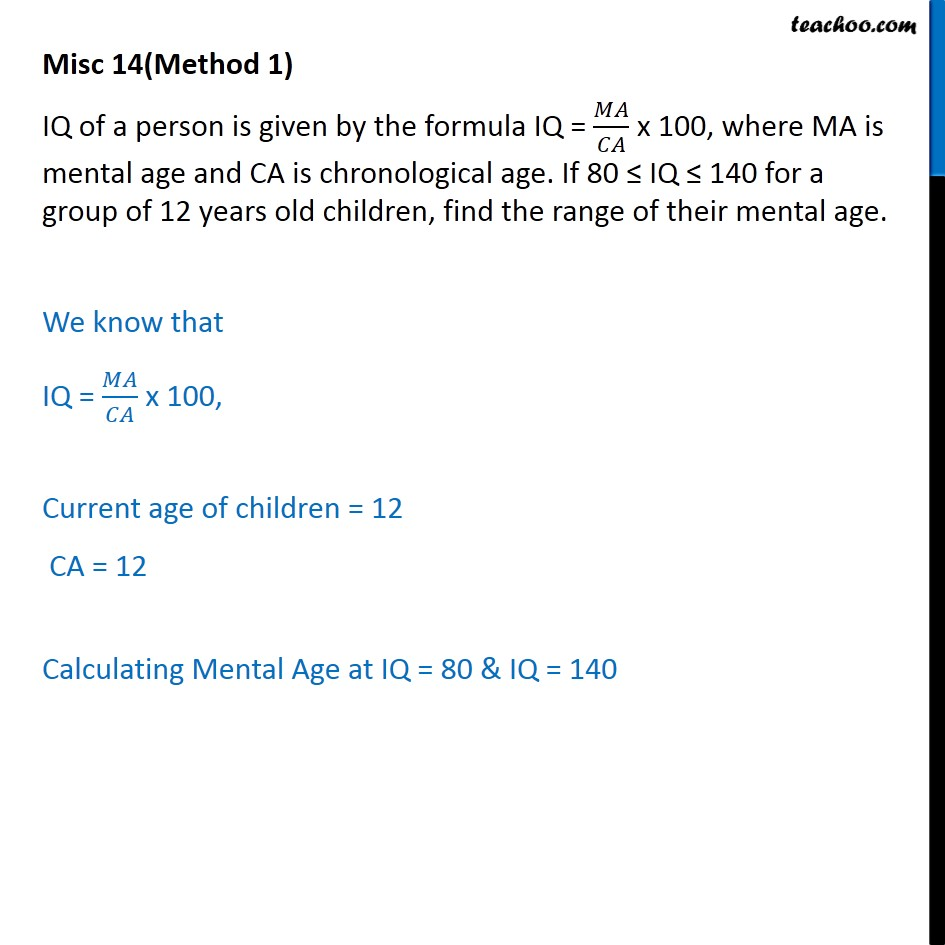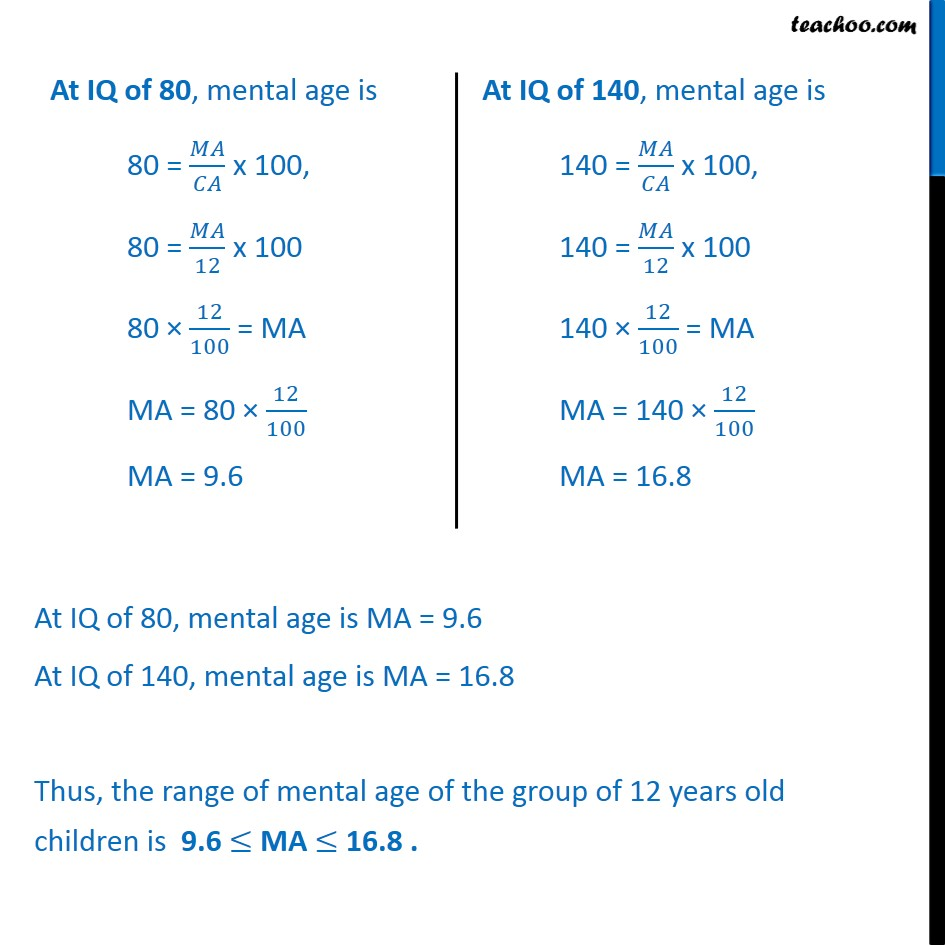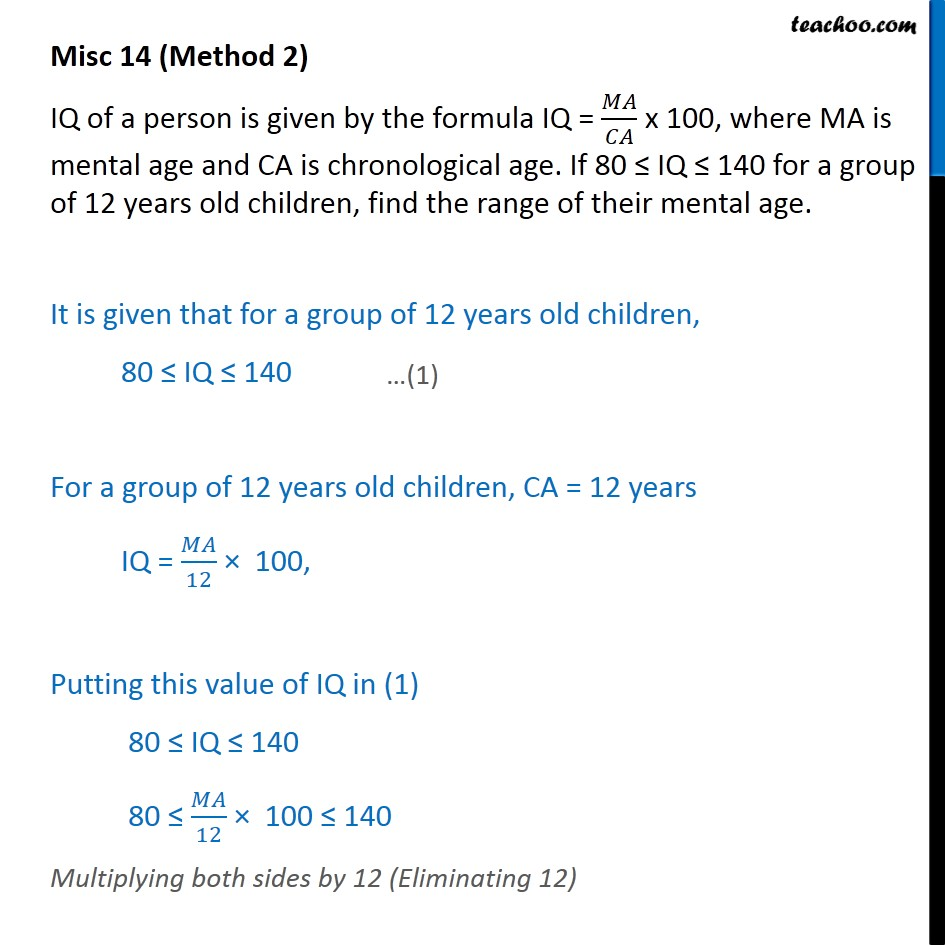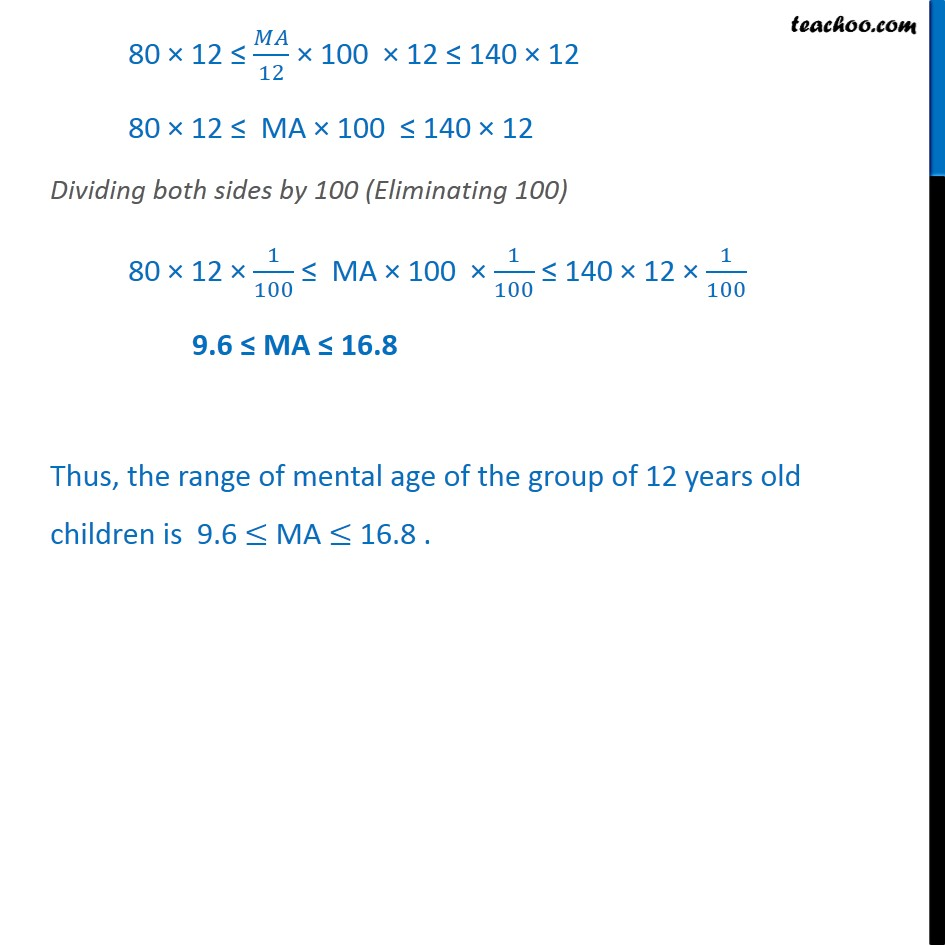Miscellaneous

Chapter 5 Class 11 Linear Inequalities
Serial order wiseLearn in your speed, with individual attention - Teachoo Maths 1-on-1 Class

### Transcript

Misc 14(Method 1) IQ of a person is given by the formula IQ = 𝑀𝐴﷮𝐶𝐴﷯ x 100, where MA is mental age and CA is chronological age. If 80 ≤ IQ ≤ 140 for a group of 12 years old children, find the range of their mental age. We know that IQ = 𝑀𝐴﷮𝐶𝐴﷯ x 100, Current age of children = 12 CA = 12 Calculating Mental Age at IQ = 80 & IQ = 140 At IQ of 80, mental age is MA = 9.6 At IQ of 140, mental age is MA = 16.8 Thus, the range of mental age of the group of 12 years old children is 9.6 ≤ MA ≤ 16.8 . Misc 14 (Method 2) IQ of a person is given by the formula IQ = 𝑀𝐴﷮𝐶𝐴﷯ x 100, where MA is mental age and CA is chronological age. If 80 ≤ IQ ≤ 140 for a group of 12 years old children, find the range of their mental age. It is given that for a group of 12 years old children, 80 ≤ IQ ≤ 140 For a group of 12 years old children, CA = 12 years IQ = 𝑀𝐴﷮12﷯ × 100, Putting this value of IQ in (1) 80 ≤ IQ ≤ 140 80 ≤ 𝑀𝐴﷮12﷯ × 100 ≤ 140 Multiplying both sides by 12 (Eliminating 12) 80 × 12 ≤ 𝑀𝐴﷮12﷯ × 100 × 12 ≤ 140 × 12 80 × 12 ≤ MA × 100 ≤ 140 × 12 Dividing both sides by 100 (Eliminating 100) 80 × 12 × 1﷮100﷯ ≤ MA × 100 × 1﷮100﷯ ≤ 140 × 12 × 1﷮100﷯ 9.6 ≤ MA ≤ 16.8 Thus, the range of mental age of the group of 12 years old children is 9.6 ≤ MA ≤ 16.8 .